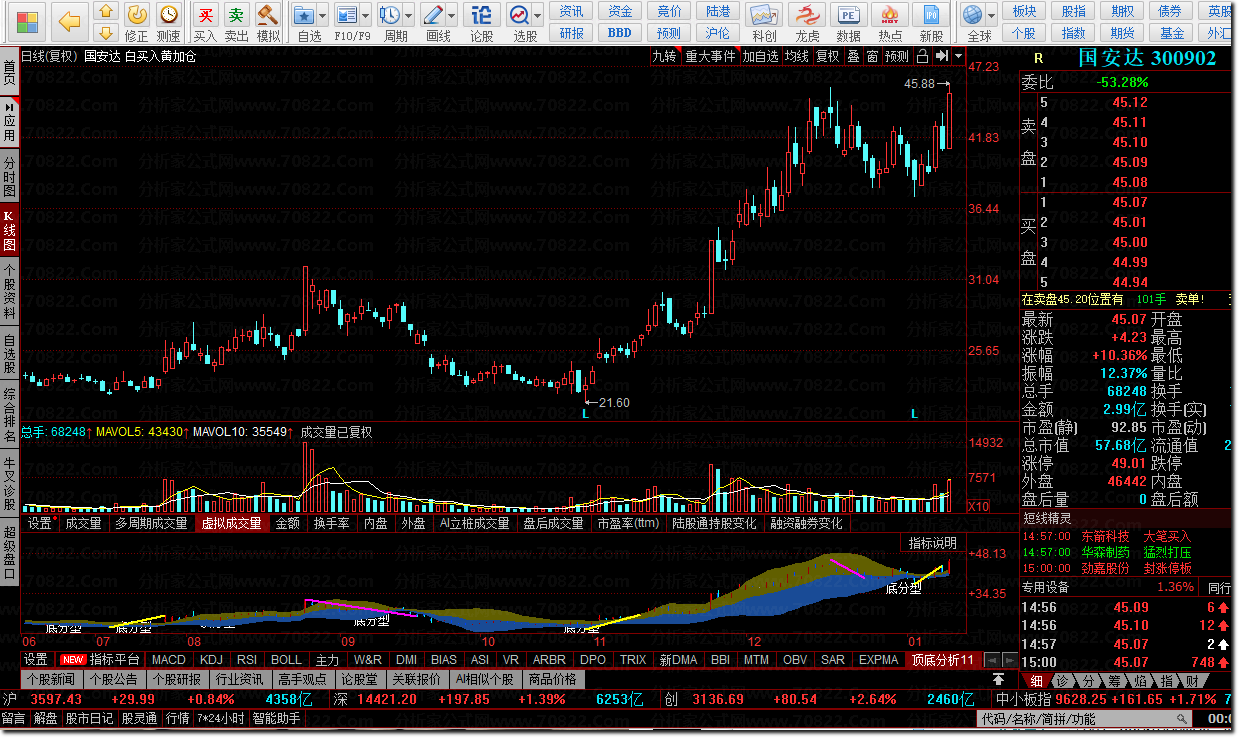• 软件大小：9.00 KB
• 推荐星级：
• 更新时间：2022-01-13 00:15:37
• 软件类别： 国产软件 / 同花顺公式
• 软件语言：简体中文
• 授权方式： 免费版
• 联系方式：暂无联系方式
• 点击大图：  【一键转帖到论坛】
• 插件情况：• 运行环境：Win10/Win9X/Win8/Win2000/WinXP/Win2003/Win7/
• 相关Tags：顶底分析
• (0)0%
(0)0%

软件介绍

DRAWBAND(BBI,RGB(97,95,00),顶,RGB(97,95,00));
DRAWBAND(BBI,RGB(25,75,150),底,RGB(25,75,250));
KU1:=IF(HIGH=HHV(HIGH,3),1,0);
KD1:=IF(LOW=LLV(LOW,3),1,0);
UL:=IF(REF(KU1,2)=1 AND REF(KU1,1)=0
AND KU1=0,REF(HIGH,2),REF(HIGH,2+BARSLAST(REF(KU1,2)=1
AND REF(KU1,1)=0 AND KU1=0)));
DL:=IF(REF(KD1,2)=1 AND REF(KD1,1)=0
AND KD1=0,REF(LOW,2),REF(LOW,2+BARSLAST(REF(KD1,2)=1
AND REF(KD1,1)=0 AND KD1=0)));
HV:=H>UL AND H>REF(H,1) ;
LV:=L<DL AND L<REF(L,1) ;
GP:=BARSLAST(HV);
DP:=BARSLAST(LV);
IP:=IF(GP=0,DP,GP)>3;
HHV1:=HV AND IP AND H>=HHV(H,BARSLAST(LV));
LV1:=LV AND IP AND L<=LLV(L,BARSLAST(HV));
DRAWLINE(LV1,LOW,HHV1, HIGH,0),coloryellow,LINETHICK2;
DRAWLINE(HHV1,HIGH,LV1, LOW,0),colormagenta,LINETHICK2;
STICKLINE(CLOSE> REF(CLOSE,1) ,HIGH,LOW,0,1 ),colorred;
STICKLINE(CLOSE> REF(CLOSE,1) ,OPEN,CLOSE,3,0 ),COLOR000055;
STICKLINE(CLOSE> REF(CLOSE,1) ,OPEN,CLOSE,2.7,0 ),COLOREEEEEE;
STICKLINE(CLOSE> REF(CLOSE,1) ,OPEN,CLOSE,2.1,0 ),COLOR000099;
STICKLINE(CLOSE> REF(CLOSE,1) ,OPEN,CLOSE,1.5,0 ),COLOR0000BB;
STICKLINE(CLOSE> REF(CLOSE,1) ,OPEN,CLOSE,0.9,0 ),COLOR0000DD;
STICKLINE(CLOSE> REF(CLOSE,1) ,OPEN,CLOSE,0.3,0 ),COLOR0000FF;
STICKLINE(CLOSE= REF(CLOSE,1) ,HIGH,LOW,0,1 ),colorwhite;
STICKLINE(CLOSE= REF(CLOSE,1) ,OPEN,CLOSE,3,0 ),COLOR555555;
STICKLINE(CLOSE= REF(CLOSE,1) ,OPEN,CLOSE,2.7,0 ),COLOR777777;
STICKLINE(CLOSE= REF(CLOSE,1) ,OPEN,CLOSE,2.1,0 ),COLOR999999;
STICKLINE(CLOSE= REF(CLOSE,1) ,OPEN,CLOSE,1.5,0 ),COLORBBBBBB;
STICKLINE(CLOSE= REF(CLOSE,1) ,OPEN,CLOSE,0.9,0 ),COLORDDDDDD;
STICKLINE(CLOSE= REF(CLOSE,1) ,OPEN,CLOSE,0.3,0 ),COLORFFFFFF;
STICKLINE(CLOSE< REF(CLOSE,1) ,HIGH,LOW,0,1 ),colorcyan;
STICKLINE(CLOSE< REF(CLOSE,1) ,OPEN,CLOSE,3,0 ),COLOR990000;
STICKLINE(CLOSE< REF(CLOSE,1) ,OPEN,CLOSE,2.7,0 ),COLORCC0000;
STICKLINE(CLOSE< REF(CLOSE,1) ,OPEN,CLOSE,2.1,0 ),COLORFF4400;
STICKLINE(CLOSE< REF(CLOSE,1) ,OPEN,CLOSE,1.5,0 ),COLORFF8800;
STICKLINE(CLOSE< REF(CLOSE,1) ,OPEN,CLOSE,0.9,0 ),COLORFFCC00;
STICKLINE(CLOSE< REF(CLOSE,1) ,OPEN,CLOSE,0.3,0 ),colorcyan;
V00:= HIGH<REF(HIGH,1) AND LOW<REF(LOW,1);
V01:= HIGH<REF(HIGH,1) AND LOW>REF(LOW,1);
V02:= HIGH>REF(HIGH,1) AND LOW<REF(LOW,1);
V03:= HIGH>REF(HIGH,1) AND LOW>REF(LOW,1);
V04:= REF(HIGH,2) < HIGH AND REF(LOW,2) < LOW
AND REF(HIGH,2) > REF(HIGH,1) AND REF(LOW,2) < REF(LOW,1);
V1:= COUNT(V00,6)>=3 AND COUNT(V01 OR V02,5)=0 AND LLVBARS(LOW,6) = 1 AND HHVBARS(HIGH,6) >= 5 AND V03;
V2:= COUNT(V00,7)>=3 AND COUNT(V01 OR V02,6)=1 AND LLVBARS(LOW,7) = 2 AND HHVBARS(HIGH,7) >= 6 AND V04;
V3:= COUNT(V00,7)>=3 AND COUNT(V01 OR V02,6)=1 AND LLVBARS(LOW,7) = 1 AND HHVBARS(HIGH,7) >= 6 AND V03;
V4:= COUNT(V00,8)>=3 AND COUNT(V01 OR V02,7)<=2 AND LLVBARS(LOW,8) = 2 AND HHVBARS(HIGH,8) >= 7 AND V04;
V5:= COUNT(V00,8)>=3 AND COUNT(V01 OR V02,7)<=2 AND LLVBARS(LOW,8) = 1 AND HHVBARS(HIGH,8) >= 7 AND V03;
V6:= COUNT(V00,9)>=3 AND COUNT(V01 OR V02,8)<=3 AND LLVBARS(LOW,9) = 2 AND HHVBARS(HIGH,9) >= 8 AND V04;
V7:= COUNT(V00,9)>=3 AND COUNT(V01 OR V02,8)<=3 AND LLVBARS(LOW,9) = 1 AND HHVBARS(HIGH,9) >= 8 AND V03;
V8:= COUNT(V00,10)>=3 AND COUNT(V01 OR V02,9)<=4 AND LLVBARS(LOW,10) = 2 AND HHVBARS(HIGH,10) >= 9 AND V04;
V9:= COUNT(V00,10)>=3 AND COUNT(V01 OR V02,9)<=4 AND LLVBARS(LOW,10) = 1 AND HHVBARS(HIGH,10) >= 9 AND V03;
V10:= COUNT(V00,11)>=3 AND COUNT(V01 OR V02,10)<=5 AND LLVBARS(LOW,11) = 2 AND HHVBARS(HIGH,11) >= 10 AND V04;
XG:= V1 OR V2 OR V3 OR V4 OR V5 OR V6 OR V7 OR V8 OR V9 OR V10;
DRAWTEXT(FILTER(XG=1,5),L*0.97,'底分型');
HV00:= HIGH>REF(HIGH,1) AND LOW>REF(LOW,1);
HV01:= HIGH<REF(HIGH,1) AND LOW>REF(LOW,1);
HV02:= HIGH>REF(HIGH,1) AND LOW<REF(LOW,1);
HV03:= HIGH<REF(HIGH,1) AND LOW<REF(LOW,1);
HV04:= REF(HIGH,2) > HIGH AND REF(LOW,2) > LOW AND REF(HIGH,2) > REF(HIGH,1) AND REF(LOW,2) < REF(LOW,1);
HV1:= COUNT(HV00,6)>=3 AND COUNT(HV01 OR HV02,5)=0 AND HHVBARS(HIGH,6) = 1 AND LLVBARS(LOW,6) >= 5 AND HV03;
HV2:= COUNT(HV00,7)>=3 AND COUNT(HV01 OR HV02,6)=1 AND HHVBARS(HIGH,7) = 2 AND LLVBARS(LOW,7) >= 6 AND HV04;
HV3:= COUNT(HV00,7)>=3 AND COUNT(HV01 OR HV02,6)=1 AND HHVBARS(HIGH,7) = 1 AND LLVBARS(LOW,7) >= 6 AND HV03;
HV4:= COUNT(HV00,8)>=3 AND COUNT(HV01 OR HV02,7)<=2 AND HHVBARS(HIGH,8) = 2 AND LLVBARS(LOW,8) >= 7 AND HV04;
HV5:= COUNT(HV00,8)>=3 AND COUNT(HV01 OR HV02,7)<=2 AND HHVBARS(HIGH,8) = 1 AND LLVBARS(LOW,8) >= 7 AND HV03;
HV6:= COUNT(HV00,9)>=3 AND COUNT(HV01 OR HV02,8)<=3 AND HHVBARS(HIGH,9) = 2 AND LLVBARS(LOW,9) >= 8 AND HV04;
HV7:= COUNT(HV00,9)>=3 AND COUNT(HV01 OR HV02,8)<=3 AND HHVBARS(HIGH,9) = 1 AND LLVBARS(LOW,9) >= 8 AND HV03;
HV8:= COUNT(HV00,10)>=3 AND COUNT(HV01 OR HV02,9)<=4 AND HHVBARS(HIGH,10) = 2 AND LLVBARS(LOW,10) >= 9 AND HV04;
HV9:= COUNT(HV00,10)>=3 AND COUNT(HV01 OR HV02,9)<=4 AND HHVBARS(HIGH,10) = 1 AND LLVBARS(LOW,10) >= 9 AND HV03;
HV10:= COUNT(HV00,11)>=3 AND COUNT(HV01 OR HV02,10)<=5 AND HHVBARS(HIGH,11) = 2 AND LLVBARS(LOW,11) >= 10 AND HV04;
HXG:=HV1 OR HV2 OR HV3 OR HV4 OR HV5 OR HV6 OR HV7 OR HV8 OR HV9 OR HV10;
DRAWTEXT(FILTER(HXG=1,3),H*1.02,'顶分型');
DRAWKLINE;软件评论评论内容只代表网友观点，与本站立场无关！

评论摘要(共 0 条，得分 0 分，平均 0 分) 查看完整评论

下载说明

* 下载方法：点击“本地高速下载”，打开新页面，如扣分0分，点击：立即下载。如扣分不是0，请注册并充分值后再点：立即下载

* 本网站提供的各种股票软件，例如大智慧软件，通达信软件，同花顺软件，飞狐，文华期货软件，手机炒股软件，指南针，东方财富通等等，和各种股票公式指标，例如大智慧公式，通达信公式，同花顺公式，操盘手公式，文华公式，飞狐公式，博易大师公式，股票价格计算公式等等公式指标，还有各类股票书籍都来源网上可以免费下载

/span> * alg格式飞狐股票公式，可以用飞狐交易师或者交易师软件导入；
* fnc格式大智慧新一代公式指标，可以用大智慧股票软件使用，少部分可以用分析家股票软件引入使用；
* exp格式大智慧经典版股票公式，仅可以用大智慧经典版股票软件引入使用；
* tni和tnc格式通达信股票公式，仅可以用通达信新引入使用，例如可以用通达信股票软件引入使用；
* tne，tn6格式通达信公式，可以用通达信公式编辑器5.0版导入，推荐通达信金融终端版本；
* hxf格式同花顺股票公式，仅可以用同花顺股票软件引入使用。

* 关于股票公式时间限制,如果在引入大智慧公式，交易师公式或者飞狐公式的时候，发现公式名称栏是空白的，这时候调整电脑时间到1997年，又能出现公式名称，并且能正常显示，可能是公式使用期限已过。
* 关于还原公式源码如果你忘记了自己编写的大智慧公式，通达信公式，同花顺公式,操盘手公式,飞狐公式，博易大师公式，文华公式和外汇EA等等公式的密码，本网可帮恢复源码，完全加密定向加密均可，收fei服务无意勿扰，点击右侧在线咨询联系我。

* 关于股票公式源码编辑

* 如果您发现下载链接错误，请点击报告错误谢谢！
* 站内提供的所有软件包含源码均是由网上搜集，若侵犯了你的版权利益，敬请来信通知我们!